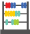# Denominator - Definition with Examples

The Complete K-5 Math Learning Program Built for Your Child

• 30 Million Kids

Loved by kids and parent worldwide

• 50,000 Schools

Trusted by teachers across schools

• Comprehensive Curriculum

Aligned to Common Core

##Let's learn!

What is a denominator?
In math, a denominator can be defined as the bottom number in a fraction that shows the number of equal parts an item is divided into.
It is the divisor of a fraction.

Here, for instance, 4 is the denominator, meaning there are 4 parts altogether.Fun Facts A denominator can never be zero!

##Let's sing!

Denominator is the number under the line,
That tells the total pie pieces when we dine!

##Let's do it!

While dividing food portions at home, ask your children to identify the denominator of the fraction that can be formed, for instance, while cutting cake or pizza in equal pieces, or while diving the greens such as beans or asparagus at the dinner table.

##Related math vocabulary

Won Numerous Awards & Honors